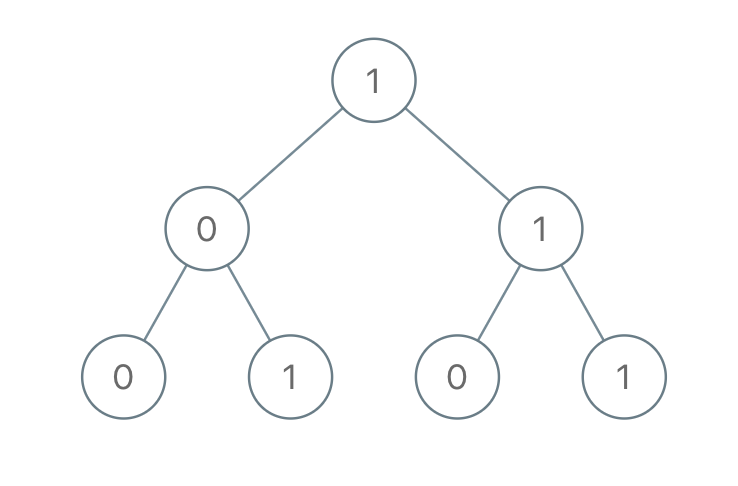1022. Sum of Root To Leaf Binary Numbers
Easy
3.1K
176

You are given the `root` of a binary tree where each node has a value `0` or `1`. Each root-to-leaf path represents a binary number starting with the most significant bit.

• For example, if the path is `0 -> 1 -> 1 -> 0 -> 1`, then this could represent `01101` in binary, which is `13`.

For all leaves in the tree, consider the numbers represented by the path from the root to that leaf. Return the sum of these numbers.

The test cases are generated so that the answer fits in a 32-bits integer.

Example 1:```Input: root = [1,0,1,0,1,0,1]
Output: 22
Explanation: (100) + (101) + (110) + (111) = 4 + 5 + 6 + 7 = 22
```

Example 2:

```Input: root = 
Output: 0
```

Constraints:

• The number of nodes in the tree is in the range `[1, 1000]`.
• `Node.val` is `0` or `1`.
Accepted
189.8K
Submissions
258.3K
Acceptance Rate
73.5%

Seen this question in a real interview before?
1/4
Yes
No

Discussion (0)

Related Topics